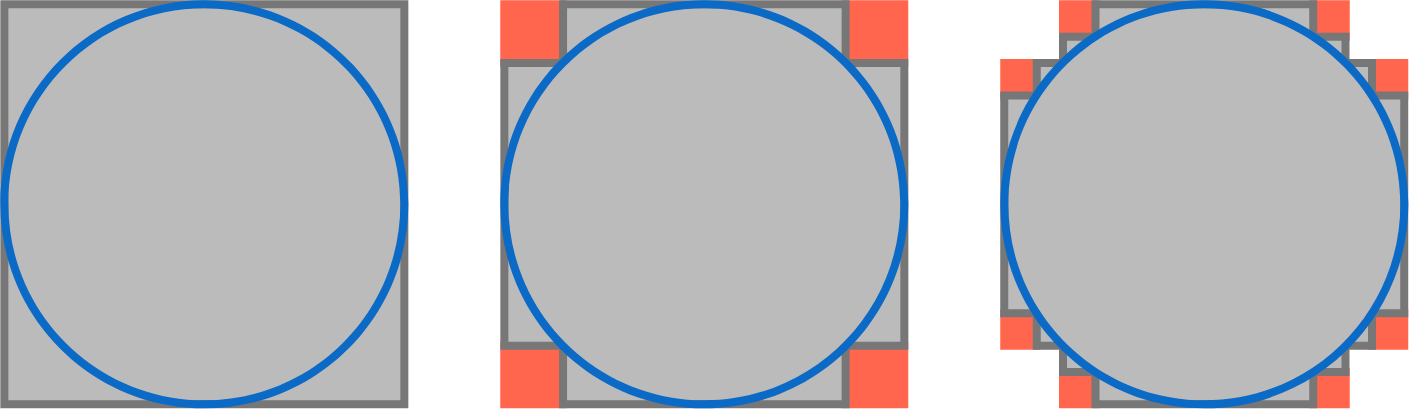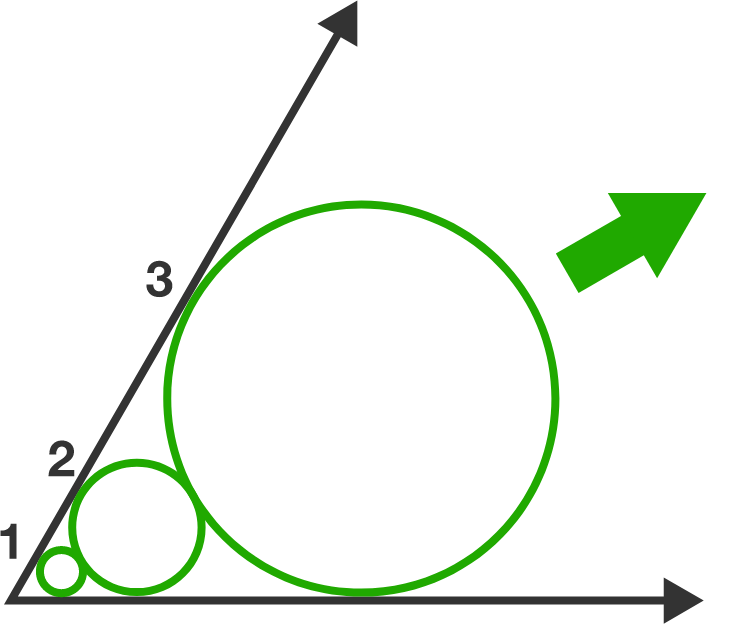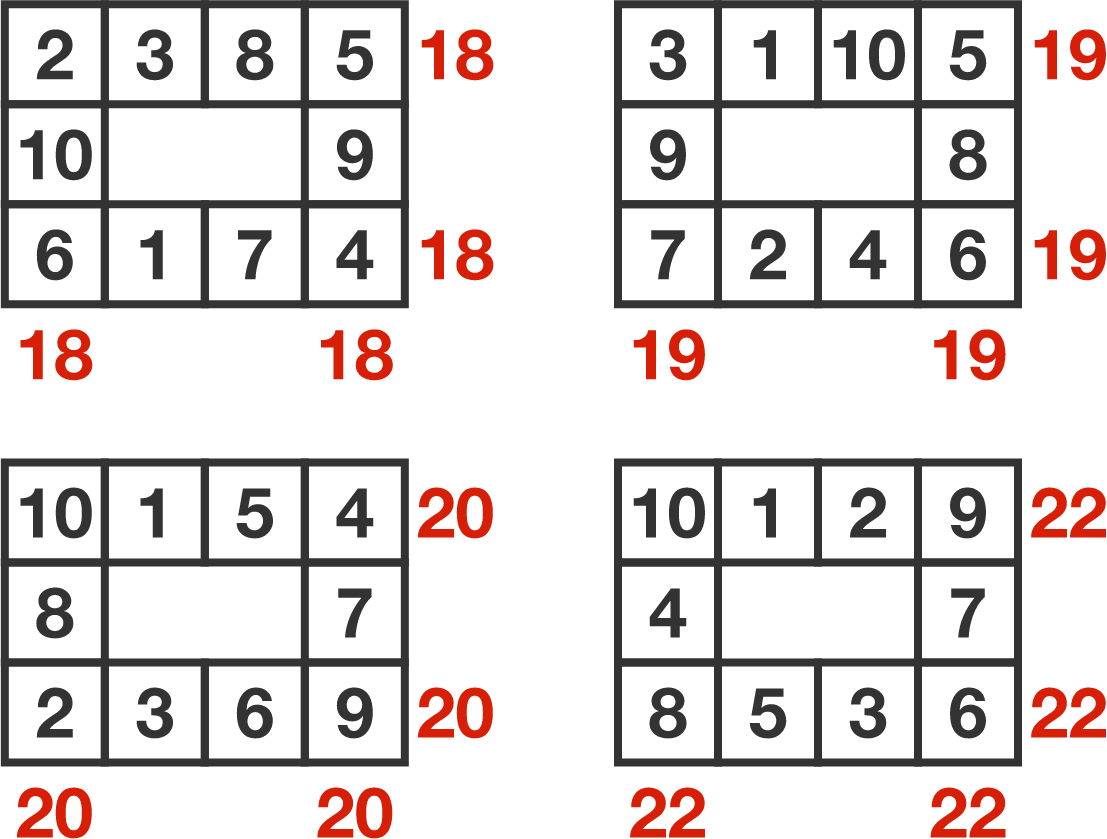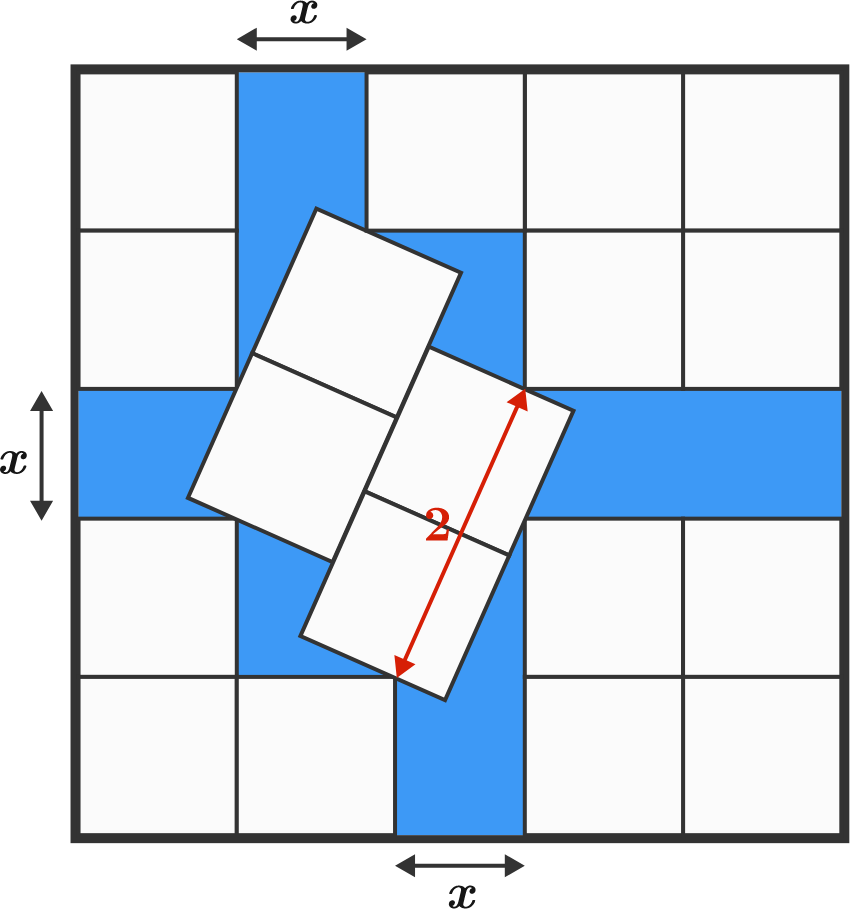# Problems of the Week

Contribute a problem

# 2018-04-09 IntermediateEmma starts with a blue circle drawn on a grey piece of square paper. She cuts out maximal squares from the corner regions that touch the circle (as indicated in red).

Which is/are correct?

A. The area of the grey region is approaching the area of the circle.
B. The length of the perimeter of the grey region is approaching the circumference of the circle.

$\large\frac{1}{2!}+\frac{2}{3!}+\frac{3}{4!}+\frac{4}{5!}+\cdots= \ ?$The two rays in the diagram make an angle of $60^\circ.$ The first circle is tangent to both rays and has radius 1. Each successive circle is tangent to the two rays and externally tangent to the previous circle.

The radius of the 2018th is $a^b$, where $a$ and $b$ are positive integers with $b$ maximized.

What is $a+b$?Each of the $3 \times 4$ tables is filled with the numbers 1 through 10 (each used exactly once) so that the sums along each outer row and column are the same number for each table. It is possible to obtain sums of 18, 19, 20, and 22.

Filling out another $3 \times 4$ table in a similar way, is it possible to obtain a sum of 21?

There are 18 white squares of side length 1 packed inside a larger square.The shaded area is equal to $\dfrac{A\sqrt{A}}{2}-B$, where $A$ is a prime number.

Find $A+B$.

×